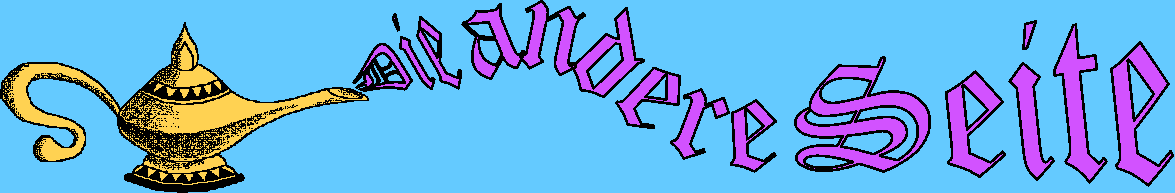GLAdapter is another way to run OpenGL /P3D. Both, Processing API and GLAdapter, use the same native libraries (JOGL) but obviously over different implementations . As Processing increased its version to 2.0 and higher my platform began to crash so I was forced to find a way to patch this bug. Let's suppose I'm not the only one with this problem so:

then unpack and install manualy

GLAdapter emulates a selected part of Processing API (P3D). It has the same names , accepts the same input params and shows the same output behavior. But the performance is not very sexy. Every usage start with this sample stub:

```

void settings(){
size(200, 200); //leave Processing in 2D
}

void setup(){
}

void draw(){

adapter.repaint();  //flush jobs, must ALWAYS be called at the end
}
```

There exist a class "GLBaseTest" which creates some test shapes.

Following functions is supported by GLAdapter:

• void lights()
• void strokeWeight(float w)
• void stroke(float r, float g, float b)
• void line(float xa, float ya, float za, float xb, float yb, float zb)
• void translate(float x, float y, float z)
• void rotateY(float angle)
• void rotateX(float angle)
• void rotateZ(float angle)
• void scale(float v)
• void beginShape(int mode) (supported mode "GLAdapter.TRIANGLE" only)
• void endShape()
• void fill(float r, float g, float b, float t)
• void fill(float c)
• void vertex(float x, float y, float z)
• void vertex(float x, float y, float z, float tx, float ty)
• void texture(PImage img)
• void background(float c)
• int color(int r, int g, int b, int a)